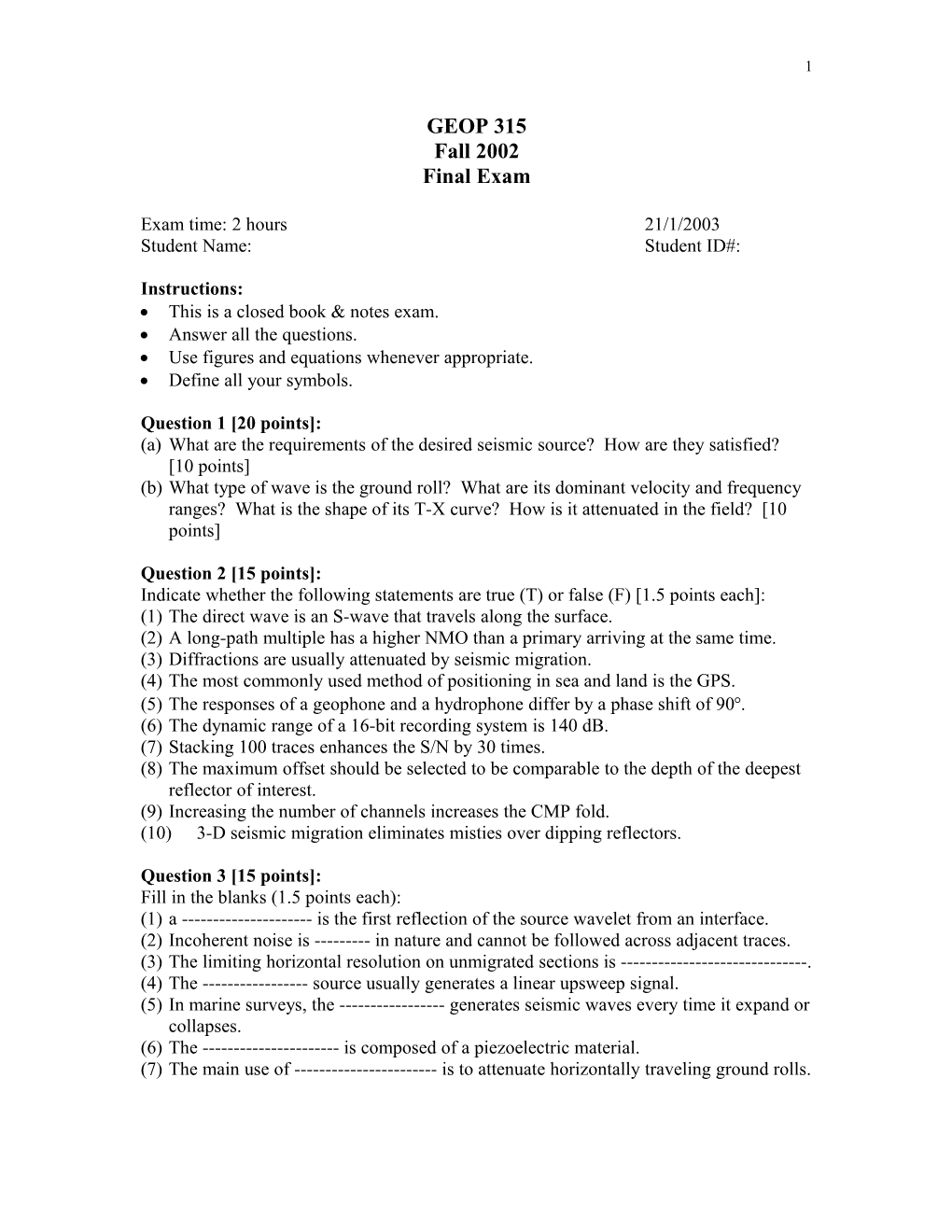# Exam Time: 2 Hours21/1/20031

GEOP 315

Fall 2002

Final Exam

Exam time: 2 hours21/1/2003

Student Name:Student ID#:

Instructions:

• This is a closed book & notes exam.
• Use figures and equations whenever appropriate.

Question 1 [20 points]:

(a)What are the requirements of the desired seismic source? How are they satisfied? [10 points]

(b)What type of wave is the ground roll? What are its dominant velocity and frequency ranges? What is the shape of its T-X curve? How is it attenuated in the field? [10 points]

Question 2 [15 points]:

Indicate whether the following statements are true (T) or false (F) [1.5 points each]:

(1)The direct wave is an S-wave that travels along the surface.

(2)A long-path multiple has a higher NMO than a primary arriving at the same time.

(3)Diffractions are usually attenuated by seismic migration.

(4)The most commonly used method of positioning in sea and land is the GPS.

(5)The responses of a geophone and a hydrophone differ by a phase shift of 90.

(6)The dynamic range of a 16-bit recording system is 140 dB.

(7)Stacking 100 traces enhances the S/N by 30 times.

(8)The maximum offset should be selected to be comparable to the depth of the deepest reflector of interest.

(9)Increasing the number of channels increases the CMP fold.

(10)3-D seismic migration eliminates misties over dipping reflectors.

Question 3 [15 points]:

Fill in the blanks (1.5 points each):

(1)a ------is the first reflection of the source wavelet from an interface.

(2)Incoherent noise is ------in nature and cannot be followed across adjacent traces.

(3)The limiting horizontal resolution on unmigrated sections is ------.

(4)The ------source usually generates a linear upsweep signal.

(5)In marine surveys, the ------generates seismic waves every time it expand or collapses.

(6)The ------is composed of a piezoelectric material.

(7)The main use of ------is to attenuate horizontally traveling ground rolls.

(8)A spread in which the source is in the center of regularly spaced geophone groups is called a ------spread.

(9)The ------is a layer near the surface, which is composed of loosely consolidated sediments.

(10)In a 3-D survey, a ------is the length over which sources are recorded without a crossline rollover.

Question 4 [15 points]

(a)What are five important factors that control the design of a 3-D survey? [7.5 points]

(b)What are five important parameters that need to be set in a 3-D survey? [7.5 points]

Question 5 [15 points]:

Figure 1 consists of several events. Answer the following questions:

(a)Indicate the direct wave and compute its velocity (V1). [3 points]

(b)Indicate the refraction and compute its velocity (V2). [3 points]

(c)Compute the critical angle (c). [3 points]

(d)Compute the thickness (H) of the first layer. [2 points]

(e)Indicate the diffraction and compute the offset and depthto the diffracting point if it lies within the first layer. [4 points]

Question 6 [20 points]:

Using the stacking chart in Figure 2, answer the following questions:

(a)What is the total number of receivers that the operator is using? [1.5 points]

(b)What is the total number of shots that the operator is using? [1.5 points]

(c)What is the number of receivers that the operator is activating with each shot? [1.5 points]

(d)What is the CDP spacing? [1.5 points]

(e)What is the spread type used with each shot? [4 points]

(f)In tables, sort the traces in:

(1)CDP domain, indicating fold for each CDP [5 points].

(2)Offset domain, indicating fold for each offset [5 points].

Figure 1

1 2 3 4 567

A XXXXXX

BXXXXXX

CXXXXXX

DXXXXXX

Spacing between stations = 20 m.

O Stands for shot location.